# Angular Momentum

## Time derivatives in a rotating frame

Let,and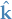be unit basis vectors in the rotating frame, with a rotational speed of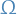about the axis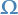. Letting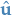be the unit vector in this frame, we have: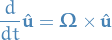Then if we have a function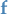, taking the derivative in the rotational frame :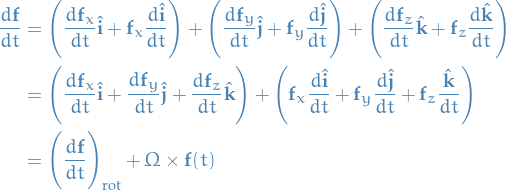Which describes the change ofwrt.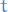using the rotational frame.

## Euler's Equations (rigid body dynamics)

From Time derivatives in a rotating frame we have: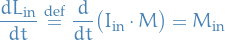where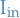is the moment of inertia tensor calculated in the intertial frame .

It's often more useful to change to coordinates to the rotating frame .

With rotating frame we mean that the coordinate axes are rotating about a fixed axis.

Why is this useful? In this frame the moment of inertia tensor is constant (and diagonal)!

## Covariance and contravariance

• Describe how the quantitive description of a certain geometric or physical entities change with a change of basis

### Covariance

• Components of a covector change in the same way as changes in scale of the reference axes → the covector and the reference axes are covariant

### Contravariance

• Components of a contr